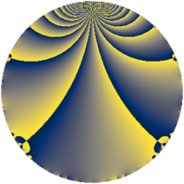Properties

 Label 1050.3.tLevel $1050$ Weight $3$ Character orbit 1050.t Rep. character $\chi_{1050}(401,\cdot)$ Character field $\Q(\zeta_{6})$ Dimension $204$ Sturm bound $720$

Related objects

Defining parameters

 Level: $$N$$ $$=$$ $$1050 = 2 \cdot 3 \cdot 5^{2} \cdot 7$$ Weight: $$k$$ $$=$$ $$3$$ Character orbit: $$[\chi]$$ $$=$$ 1050.t (of order $$6$$ and degree $$2$$) Character conductor: $$\operatorname{cond}(\chi)$$ $$=$$ $$21$$ Character field: $$\Q(\zeta_{6})$$ Sturm bound: $$720$$

Dimensions

The following table gives the dimensions of various subspaces of $$M_{3}(1050, [\chi])$$.

Total New Old
Modular forms 1008 204 804
Cusp forms 912 204 708
Eisenstein series 96 0 96

Trace form

 $$204 q + 204 q^{4} - 8 q^{6} - 6 q^{7} - 4 q^{9} + O(q^{10})$$ $$204 q + 204 q^{4} - 8 q^{6} - 6 q^{7} - 4 q^{9} - 68 q^{13} - 408 q^{16} + 8 q^{18} + 62 q^{19} - 6 q^{21} + 16 q^{22} - 8 q^{24} - 132 q^{27} + 130 q^{31} - 126 q^{33} - 16 q^{34} - 16 q^{36} + 70 q^{37} + 50 q^{39} + 16 q^{42} + 20 q^{43} - 48 q^{46} - 126 q^{49} - 114 q^{51} - 68 q^{52} - 104 q^{54} - 56 q^{57} - 16 q^{58} - 356 q^{61} + 48 q^{63} - 1632 q^{64} + 64 q^{66} - 6 q^{67} + 332 q^{69} - 16 q^{72} + 150 q^{73} + 248 q^{76} - 32 q^{78} + 194 q^{79} + 176 q^{81} - 176 q^{82} + 48 q^{84} + 488 q^{87} + 16 q^{88} + 98 q^{91} + 20 q^{93} + 480 q^{94} + 16 q^{96} + 600 q^{97} - 316 q^{99} + O(q^{100})$$

Decomposition of $$S_{3}^{\mathrm{new}}(1050, [\chi])$$ into newform subspaces

The newforms in this space have not yet been added to the LMFDB.

Decomposition of $$S_{3}^{\mathrm{old}}(1050, [\chi])$$ into lower level spaces

$$S_{3}^{\mathrm{old}}(1050, [\chi]) \cong$$ $$S_{3}^{\mathrm{new}}(21, [\chi])$$$$^{\oplus 6}$$$$\oplus$$$$S_{3}^{\mathrm{new}}(42, [\chi])$$$$^{\oplus 3}$$$$\oplus$$$$S_{3}^{\mathrm{new}}(105, [\chi])$$$$^{\oplus 4}$$$$\oplus$$$$S_{3}^{\mathrm{new}}(210, [\chi])$$$$^{\oplus 2}$$$$\oplus$$$$S_{3}^{\mathrm{new}}(525, [\chi])$$$$^{\oplus 2}$$